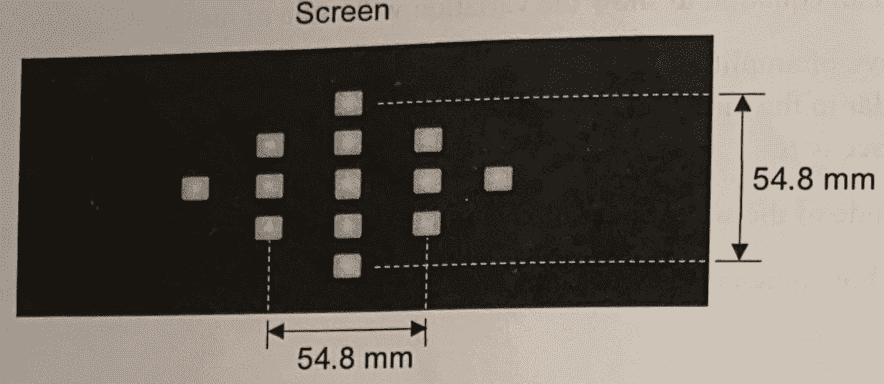# Determine horizontal separation of fabric from diffraction pattern

songoku
Homework Statement:
When a laser of 684 nm wavelength is incident on a fabric, a diffraction pattern is observed on a screen placed at a distance of 2 m away as shown below. Determine the separation between horizontal threads of the fabric
Relevant Equations:
d sin = n λDo I need to use formula to answer this question? Can't I just divided the horizontal distance in the picture by 2. so the horizontal separation of the thread is 54.8 / 2 = 27.4 mm?

Thanks

Homework Helper
Gold Member
2022 Award
Do I need to use formula to answer this question? Can't I just divided the horizontal distance in the picture by 2. so the horizontal separation of the thread is 54.8 / 2 = 27.4 mm?

Thanks
It's a diffraction pattern, not a simple projection. Yes, you need to use the formula.

•songoku
songoku
It's a diffraction pattern, not a simple projection. Yes, you need to use the formula.
Is formula for horizontal separation the same as vertical distance? What I learned so far is all about vertical distance measured from central maxima.

My attempt:
d sin θ = nλ and assuming θ is small, then sin θ = y / L where y is the horizontal distance measured from central maxima and L is distance between screen and the fabric.

Taking n = 1 :
d sin θ = nλ
d y / L = λ
d = L . λ / y = 2 (684 x 10-9 / (27.4 x 10-3) = 5 x 10-5 m

Is this correct? Thanks

Last edited:
Homework Helper
Gold Member
2022 Award
Is formula for horizontal separation the same as vertical projection? What I learned so far is all about vertical distance measured from central maxima.

My attempt:
d sin θ = nλ and assuming θ is small, then sin θ = y / L where y is the horizontal distance measured from central maxima and L is distance between screen and the fabric.

Taking n = 1 :
d sin θ = nλ
d y / L = λ
d = L . λ / y = 2 (684 x 10-9 / (27.4 x 10-3) = 5 x 10-5 m

Is this correct? Thanks
Looks right.

•songoku
songoku
Thank you very much haruspex